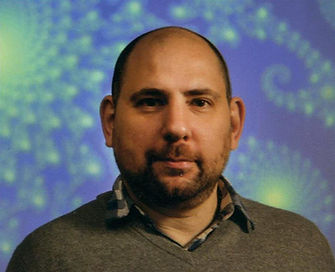top of page

## Pablo ShmerkinI am a Professor at the University of British Columbia (on leave from T. Di Tella University). My pronouns are he/him.

My work generally spans the areas of fractal geometry, analysis, and ergodic theory, although I am also interested in connections between these fields and  probability and combinatorics.

In particular, my recent work is concerned with:

• Combinatorial problems in fractal geometry (projection theory, distance sets, Furstenberg set problem, etc).

• Geometric properties of (random and deterministic) fractals of dynamical, arithmetic and combinatorial origin. In particular, geometrical aspects of dynamical rigidity and smoothness of self-similar measures.

• Applications of fractal geometry in ergodic theory and analysis.

## RECENT ARTICLES

• Tuomas Orponen, Nicolas de Saxcé and Pablo Shmerkin. On the Fourier decay of multiplicative convolutions. Arxiv .

• Pablo Shmerkin. Inverse theorems for discretized sums and \$L^q\$ norms of convolutions in \$\mathbb{R}^d\$. Arxiv .

• Tuomas Orponen and Pablo Shmerkin. Projections, Furstenberg sets, and the ABC sum-product problem. Arxiv .

• Pablo Shmerkin and Hong Wang. Dimensions of Furstenberg sets and an extension of Bourgain's projection theorem. Arxiv .

• Tuomas Orponen, Pablo Shmerkin and Hong Wang. Kaufman and Falconer estimates for radial projections and a continuum version of Beck's Theorem. Arxiv.

bottom of page